Courses

# Short & Long Answer Question(Part-2)- Magnetic Effects of Current Class 10 Notes | EduRev

## Class 10 : Short & Long Answer Question(Part-2)- Magnetic Effects of Current Class 10 Notes | EduRev

The document Short & Long Answer Question(Part-2)- Magnetic Effects of Current Class 10 Notes | EduRev is a part of the Class 10 Course Science Class 10.
All you need of Class 10 at this link: Class 10

Ques 1: How will you find out the direction of the magnetic field produced by current-carrying conductor?
Ans: The direction of lines of force of the magnetic field produced by a straight wire carrying current is obtained by Maxwell’s right hand thumb rule. According to Maxwell’s right-hand thumb rule, “Imagine that the current carrying wire is in the right hand so that the thumb points in the direction of current, then the direction in which the fingers encircle the wire gives the direction of magnetic lines of force around the wire.
Imagine a current carrying wire AB in which the current is flows vertically upwards. To find out the direction of magnetic lines of force produced by this current, we imagine the wire AB to be held in the right hand, so that the thumb points in the direction of current towards A. Now, the direction in which the fingers are folded gives the direction of the lines of force. In this case the fingers are folded in the anti-clockwise direction, so the magnetic lines of force are also in the anti-clockwise direction.

Ques 2: What type of core should be put inside a current-carrying solenoid to make an electromagnet?
Ans: A soft iron core is placed inside a solenoid to make an electromagnet. When a soft iron core is placed inside a solenoid, then the strength of the magnetic field becomes very large because the iron core gets magnetized by induction. This combination of a solenoid and a soft iron core is called an electromagnet.

Ques 3: Distinguish between a bar magnet and an electromagnet.
Ans: Bar Magnets
The bar magnet is a permanent magnet.
It produces a comparatively weak force of attraction.
The strength of a bar magnet cannot be changed.
The polarity of a bar magnet is fixed and cannot be changed.
Electromagnets
An electromagnet is a temporary magnet.
It produces a very strong magnetic force.
The strength of an electromagnet can be changed by changing the number of turns in its coil or by changing the current passing through it.
The polarity of an electromagnet can be changed by changing the direction of current in its coil.

Ques 4: State the composition of the alloy called nipermag? Give an important use of this alloy.
Ans: Nipermag is an alloy of iron, nickel, aluminium and titanium. Permanent magnet of this alloy is more stronger than those made of ordinary steel. Hence it used in microphones and loudspeakers.

Ques 5: Derive the formula for the force acting on a charged particle moving in a magnetic field.
Ans: The force acting on a current-carrying conductor placed in a magnetic field is,
F = B × I × L
The current I is the rate of flow of charge.
Now, if a charge Q flows in time t then the current I = Q/t. Hence substituting for I in
the above equation, we get,
F = (B × Q × L)/t
Suppose the particle carrying the charge Q travels a length L in time t, then the velocity
v = L/t. So substituting this value, we get
Force on moving charge F = B × Q × v.

Ques 6: How does alternating current differ from the direct current?
Ans: If current always flows in the same direction, it is called a direct current. The current, which we get from the cell or a battery, is a direct current because it always flows in the same direction. The positive and negative polarity of a direct current is fixed. If the current changes direction after regular intervals of time, it is called alternating current. Most of the power stations in India generate alternating current. The alternating current produced in India changes its direction every 1/100 second. Thus, the positive and negative polarity of an alternating current is not fixed.

Ques 7: Give two reasons why different electrical appliances in a domestic circuit are connected in parallel.
Ans: (i) If one of the appliances is switched off or gets fused, there is no effect on the other appliances and they keep on operating.
(ii) The same voltage of the main line is available for all electrical appliances.

Ques 8: Why is a fuse wire made of a tin-lead alloy and not copper?
Ans: A fuse wire is made of tin alloy because it has low melting point, so that it may melt easily, whereas a copper wire cannot be used as a fuse wire because it has a high melting point due to which it will not melt easily when a short circuit takes place.

Ques 9: Explain the principle and working of an electric motor with the help of a diagram. What is the function of a split ring commutator?
Ans: An electric motor converts electrical energy into mechanical energy. It works on the principle that – a current carrying conductor placed in a magnetic field experiences a force.
Following are the essential parts of an electric motor.
(i) Coil: It is a rectangular coil of insulated copper wire having large number of turns.
(ii) A large permanent magnet provides strong magnetic field between its pole pieces. The coil rotates between these pole pieces.
(iii) Split rings: The two ends of the coil are connected to two split rings, which are two halves of a slip rings.
(iv) Brushes: Two carbon brushes keep in sliding contact with split rings.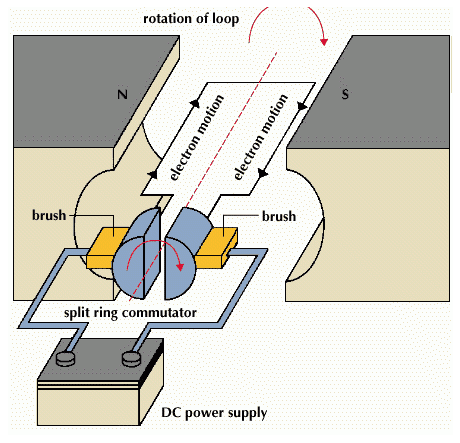Working
When a current is passed through the coil, the direction of current in AB and CD is in opposite direction but both are perpendicular to magnetic field. Therefore, by Fleming’s left hand rule AB arm of the coil experiences an upward force and CD arm experiences a downward force. These two forces being equal and opposite to each other form a couple which rotates the coil. Arms BC and DA are parallel to the field and the force on them is zero. The forces, on AB and CD turn the coil in clockwise direction. After half revolution, the split rings change their position. Now S2 is in contact with brush B1 and S1 is in contact with B2. So the direction of current in the coil reverses. Therefore, AB now experiences downward force and CD upward force. The couple now acting on the coil again moves it in clockwise direction. Due to the function of split ring commutator and brushes, coil continues to turn in clockwise direction.
Split ring commutator changes direction after every half rotation, so that the direction of current going in the coil also reverses and the arm of the coil which goes up in the first half, goes down in second half. As a result, the coil continues to rotate in one direction. Anything connected to the axis of the coil also rotates. So, the electrical energy given to the coil changes into mechanical energy.

Ques 10: With the help of a labelled diagram, explain the working of an A.C. generator.
Ans: “A. C. generator” means “Alternating Current generator”. That is, an A. C. generator produces alternating current, which alternates (changes) in polarity continuously. We will now describe the construction an working of the A. C. generator or A. C. dynamo.
Construction of an A. C. generator
A simple A. C. generator consists of a rectangular coil ABCD that can be rotated rapidly between the poles N and S of a strong horseshoe type magnet M. The coil is made of a large number of turns of insulated copper wire. The ends A and D of the rectangular coil are connected to two circular pieces of copper metal called slip rings R1 and R2. As the slip rings R1 and R2 rotate with the coil, the two pieces of carbon called brushes, B1 and B2, keep contact with them. So, the current produced in the rotating coil can be tapped out through slip rings into the carbon brushes. From the carbon brushes B1 and B2 we take the current into various electrical appliances like radio, T. V., electric iron, bulbs, etc. But in this figure, we have shown only a galvanometer G connected the two carbon brushes.
Working of an A. C. generator
Suppose that the generator coil ABCD is initially in the horizontal position. Again suppose that he coil ABCD is being rotated in the anticlockwise direction between the poles N and S of a horseshoe type magnet.
(i) As the coil rotates in the anticlockwise direction, the side AB of the coil moves down cutting the magnetic lines of force near the N-pole of the magnet, and side CD moves up, cutting the lines of force near the S-pole of the magnet. Due to this, induced current is produced in the sides AB and DC of the coil. On applying Fleming’s right hand rule to the side AB and DC of the coil, we find that the currents are in the direction B to A and D to C respectively. Thus, the induced currents in the two sides of the coil are in the same direction, and we get an effective induced current in the direction BADC.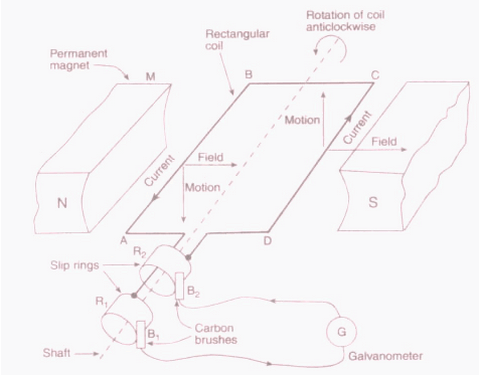(ii) After half revolution, the sides AB and DC of the coil will interchange their positions. The side AB will come on the right hand side and DC will come on the left side. So, after half a revolution, side AB starts moving up and side DC starts coming down. As a result of this, the direction of induced current in each side of the coil is reversed after half a revolution. Since the direction of induced current in the coil is reversed after half revolution so the polarity (positive and negative) of the two ends of the coil also changes after half revolution.
The end of coil which was positive in the first half of rotation becomes negative in the second in the second half. And the end which was negative in the first half revolution becomes positive in the second half of revolution. Thus, in 1 revolution of the coil, the current changes its direction 2 times.The alternating current (A. C.) produced in India has a frequency of 50 Hz.
That is, the coil is rotated at the rate of 50 revolutions per second. Since in 1 revolution of coil, the current changes its direction 2 times, so in 50 revolutions of coil, the current changes its direction 2 × 50 = 100 times. Thus, the A. C. supply in India changes its direction 100 times in 1 second. Another way of saying this is that the alternating current produced in India changes its direction every 1/100 second. That is, each terminal of the coil is positive (+) for 1/100 of a second and negative (-) for the next 1/100 of a second. This process is repeated again and again with the result that there is actually no positive and negative in an A. C. generator. We will now describe why the direction of induced current in the coil of an A. C. generator changes after every half revolution of the coil.
After every half revolution, each side of the generator coil starts moving in the opposite direction in the magnetic field. The side of the coil which was initially moving downwards in a magnetic field, after half revolution, it starts moving in opposite direction – upwards. Similarly the side of coil which was initially moving upwards, after half revolution, it starts moving downwards. Due to the change in the direction of motion of the two sides of the coil in the magnetic field after every half revolution, the direction of current produced in them also changes after every half revolution.

Ques 11: Explain the principle, construction and working of a DC Motor.
Ans: “D. C. generator” means “Direct Current generator”. That is, a D. C. generator
produces direct current and not alternating current. We will now describe the construction and working of D. C. generator or D. C. Dynamo.
Construction of a D. C. generator
A simple D. C. generator consists of a rectangular coil ABCD which cab be rotated rapidly between the poles N and S of a strong horse-shoe type magnet M. The generator coil is made of a large number of turns of insulated copper wire. The two ends of the coil are connected to the two copper half rings (or split rings) R1and R2 of a commutator. There are two carbon brushes B1 and B2 which press lightly against the two half rings. When the coil is rotated, the two half rings R1 and R2 touch the two carbon brushes B1 and B2 one by one. So the current produced in the rotating coil can be tapped out through the commutator half rings into the carbon brushes. From the carbon brushes B1 and B2, we can take the current into the various electrical appliances like radio, T. V., electric iron, bulbs, etc. But in this figure, we have shown only a galvanometer G connected between the two carbon brushes. The galvanometer is a current detecting and current measuring instrument.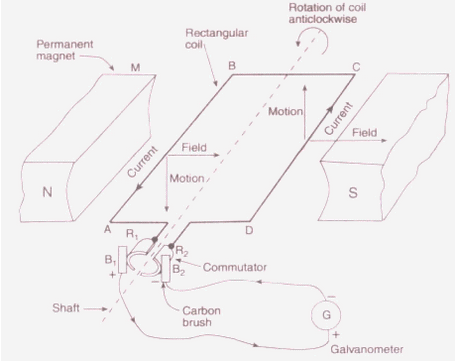Working of a D. C. generator
Suppose that the generator coil ABCD is initially in the horizontal position. Again suppose that he coil ABCD is being rotated in the anticlockwise direction between the poles N and S of a horseshoe type magnet.
As the coil rotates in the anticlockwise direction, the side AB of the coil moves down cutting the magnetic lines of force near the N-pole of the magnet, and side DC moves up, cutting the lines of force near the S-pole of the magnet. Due to this, induced current is produced in the sides AB and DC of the coil. On applying Fleming’s right hand rule to the side AB and DC of the coil we find that the currents in them are in the direction B to A and D to C respectively. Thus, the induced currents in the two sides of the coil are in the same direction, and we get an effective induced current in the direction BADC. Due to this the brush B1becomes a positive (+) pole and brush B2 becomes negative (-) pole of the generator.
After half revolution, the sides AB and DC of the coil will interchange their positions. The side AB will come on the right hand side and start moving up whereas side DC will come on then the two commutator half rings R1 and R2 automatically change their contacts from one carbon brush to the other. Due to this change, the current keeps flowing in the same direction in the other circuits. The brush B1 always remaining positive terminal and brush B2 always remaining negative terminal of the generator. Thus, a D. C. generator supplies a current in one direction by the use of a commutator consisting of two, half-rings of copper. In the above discussion we have used the word D. C. generator everywhere. Please note that we can also write D. C. dynamo in place of D. C. generator.

Ques 12: What is a fuse wire? What is the advantage and disadvantage of using a thick fuse wire?
Ans: A fuse is a very important device used for protecting electric circuits. It is a wire made out of a metal like tin or tin alloy having a very low melting point.
When a high current flows through a circuit, the fuse wire gets heated or melts due to short-circuiting or overloading. Hence the circuit is broken and the current stops flowing. This saves all the appliances of the circuit.

Ques 13: The device used for producing current is called a,
(i) Generator
(ii) Voltmeter
(iii) Ammeter
(iv) Galvanometer.

Ans: (i) Generator. The other devices are measuring instruments.

Ques 14: What are magnetic field lines? How is the direction of a magnetic field at a point determined? Mention two important properties of the magnetic field lines.
Ans: The space surrounding a magnet in which magnetic force is exerted, is called a magnetic field. Magnetic field lines are the lines that are drawn at every point indicating the direction in which a north pole would move if placed at that point. They are determined by placing an imaginary hypothetical north pole at that point and finding the direction in which it would move due to the magnetic field at that point. A compass needle gets deflected when placed near a magnet due to the magnetic force exerted by the magnet on it.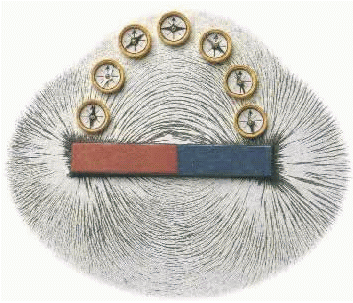Some important properties of magnetic field lines are:
(i) The tangent drawn at any point on the field line indicates the direction in which a north pole would move if placed at that point.
(ii) The relative strength of the field is proportional to the degree of closeness of the lines. The more clustered they are, the stronger the field in that region.
(iii) The magnetic field lines never intersect. This is because a pole can move only in zone direction and if the lines intersect they would have to move in two direction simultaneously which is impossible.

Ques 15: Draw a rough sketch of the pattern of field lines due to a
(i) current flowing into a circular coil and
(ii) solenoid carrying current.

Ans: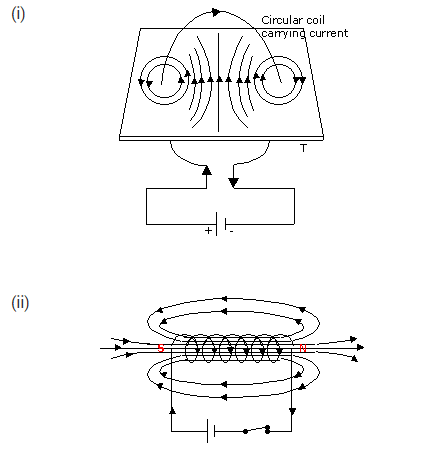Ques 16: State the rule to determine the direction of a
(i) magnetic field produced around a straight conductor-carrying current,
(ii) force experienced by a current-carrying straight conductor placed in a magnetic field which is perpendicular to it, and
(iii) current induced in a coil due to its rotation in a magnetic field.
(iv) Current induced in a circuit by the changing magnetic flux due to the motion of a magnet.

Ans: (i) The direction of a magnetic field produced around a current-carrying conductor can be obtained by using Maxwell’s right-hand thumb rule. It states that “if you hold the current carrying wire in your right hand with your thumb pointing in the direction of the magnetic field then the fingers will wrap around the conductor in the direction of the magnetic field lines due to the conductor”.
(ii) The direction of the force experienced by a straight conductor carrying current placed in a magnetic field is determined using Fleming’s left hand rule. It states that “if you stretch the forefinger, the central finger and the thumb of your left hand mutually perpendicular to each other, the forefinger points in the direction of the magnetic field and the central finger points in the direction of current, and the thumb points in the direction of force acting on the conductor”.
(iii) The direction of the current induced in a circuit by changing the magnetic flux due to motion of a conductor is given by Fleming’s right hand rule. It states that “if you hold the forefinger, the central finger and the thumb of your right hand mutually perpendicular to each other, the forefinger indicates the direction of the changing field / flux, the thumb indicates the direction of motion of the conductor and the middle finger gives the direction of the induced current”. This phenomenon is called electromagnetic induction.

Ques 17: On what factors does the force experienced by a current–carrying conductor placed in a uniform magnetic field depend?
Ans: The force on a current carrying conductor placed in a magnetic field is given by the Fleming’s left hand rule.
F = B I L
Where F is the force on the conductor
B is the magnitude of the uniform magnetic field
I is the current in the conductor
L is the Length of the current carrying wire
Therefore from the above formula, force is directly proportional to the magnitude of the field, current in the wire and the length of the wire.

Ques 18: Explain the principle and working of an electric motor with the help of a diagram.What is the function of a split–ring commutator ?
Ans: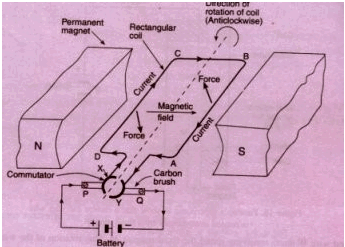A motor is a device that converts the electrical energy into mechanical energy.Principle
An electric motor is based on the fact that when a current carrying conductor is placed in a magnetic field the conductor experiences a force which is given by Fleming’s Left Hand Rule. For example, when a rectangular coil is placed in the magnetic field and current is passed through it, a torque acts on the coil, which rotates it continuously. When the coil rotates, the shaft attached to it also rotates and therefore the electrical energy supplied to the motor is converted into the mechanical energy of rotation.
An electrical motor consists of a rectangular coil ABCD of insulated copper wire, wound on a soft iron core called armature. The coil is mounted between the poles of a magnet in such a way that it can rotate between the poles N and S. The two ends of the coil are soldered to the ends of a commutator whose main function is to reverse the direction of the current flowing through the coil every time the coil just passes the vertical position during its revolution.
Working
Suppose the coil ABCD is initially at a horizontal position. When the switch is in ON position the current enters the coil through the carbon brushes and the half ring ‘A’ of the commutator.
The current flows in the direction DCBA and leaves via the half ring ‘B’. In the side PQ of the coil, the direction is from Q to P towards the south and the direction of the magnetic field is from the N to S pole towards the east. So, by applying Fleming’s left hand rule, we find that it will experience a force in upward direction. Similarly, the side SR of the coil will experience a downward force. Thus we have two parallel wires experiencing forces in opposite directions. They form a couple tending to rotate the coil in the anticlockwise direction.
When the coil goes beyond the vertical position, the two commutator half rings automatically changes contact from one brush to the other. This reverses the direction of current through the coil which, in turn, reverses the direction of forces acting on the two sides of the coil. The sides of the coil are interchanged, but rotate in the same anticlockwise direction. This process is repeated again and again and the coil continues to rotate as long as the current is passing.

Ques 19: A coil of copper wire is connected to a galvanometer. What would happen if a bar magnet is
(i) Pushed into the coil with its north pole entering first?
(ii) Pulled out of the bar magnet?
(iii) Held stationary inside the coil?

Solution: (i) A deflection is observed in the galvanometer due to the induced current because of the changing magnetic flux (increasing) through the turns of the coil connected to the galvanometer.
(ii) A deflection is again observed in the galvanometer, as when it is pulled out, the flux linked with the coil due to the bar magnet decreases. Hence a current flows in the coil to reduce the change in flux. The deflection can be observed in the opposite direction as compared with the previous case.
(iii) No deflection is observed in the galvanometer. The flux linked with the coil due to the magnetic field is at a constant. Hence no current is induced due to the bar magnet.

Que 20: Draw a labelled diagram to explain the principle underlying the working of an electric generator.
Solution: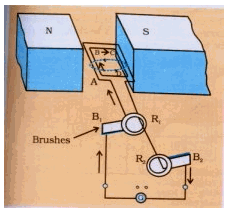Ques 21: What is the function of an earth wire? Why is it necessary to earth the metallic appliances?
Ans: To avoid electric shocks, the metal body of an electrical device is ‘earthed’. A wire called ‘earth wire’ is used to connect the metal body of the electrical device to the earth, which is at zero potential. In household circuits, we have three wires, the live wire, the neutral wire and the earth wire. One end of the earth wire is connected to the device and the other end of the wire is connected to the earth. We now say that the device is “earthed” or “grounded”. Usually the three wires are connected to a three-pin plug. The neutral wire or the earth connection carries the high current to the earth from the device and prevents an electric shock.

Ques 22: Explain what is short-circuiting and overloading in an electric supply.
Ans: Short circuiting
If the plastic insulation of the live wire and neutral wire gets torn, then the two wires touch each other. This touching of the live wire and neutral wire directly is known as short-circuiting. The current passing through the circuit formed by these wires is very large and consequently a high heating effect is created which may lead to fire.
Overloading
The current flowing in domestic wiring at a particular time depends on the power ratings of the appliances being used. If too many electrical appliances of high power rating are switched on at the same time, they draw an extremely large current from the circuit. This is known as overloading. Due to this large current flowing through them, the copper wires of household wiring get heated to a very high temperature and may lead to fire.

Ques 23: Describe an experiment to illustrate the action of an electric fuse.
Ans: Take a thin fuse wire made of tin or tin-alloy having low melting point. Place this fuse wire on the porcelain fuse grip and insert the grip into the fuse holder. Now switch on all the electrical appliances of high power rating like electric iron, water heater, air conditioner, etc.,. Since the melting point of the fuse wire is much lower, it melts and breaks the circuit.

Ques 24: Imagine that you are sitting in a chamber with your back to one wall. An electron beam, moving horizontally from back wall towards the front wall, is deflected by a strong magnetic field to your right side. What is the direction of the magnetic field?
Ans:  vertically downwards.

Offer running on EduRev: Apply code STAYHOME200 to get INR 200 off on our premium plan EduRev Infinity!

## Science Class 10

171 videos|320 docs|156 tests

,

,

,

,

,

,

,

,

,

,

,

,

,

,

,

,

,

,

,

,

,

;